Time: 3 Hours                                                                                                     Max. Marks: 100

NOTE: There are 9 Questions in all.

·      Question 1 is compulsory and carries 20 marks. Answer to Q. 1. must be written in the space provided for it in the answer book supplied and nowhere else.

·      Out of the remaining EIGHT Questions answer any FIVE Questions. Each question carries 16 marks.

·      Any required data not explicitly given, may be suitably assumed and stated.

Q.1       Choose the correct or best alternative in the following:                                         (2x10)

a.       For a rectangular wave guide having A as broader dimension and B as  narrow dimension, the cutoff wave length for TE10 mode will be

(A)  A                                                 (B)  2A

(C)   B                                                (D)  2B

b.      Magic tee is a

(A)    Modification of E plane tees          (B)  H plane tee

(C)  E-plane tee                                   (D) Combination  of E plane and H

plane tee.

c.   An air filled rectangular waveguide has dimensions 6 cm, 4 cm. The cut off frequency for TE01 mode is

(A)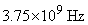(B)  2.5 GHz

(C)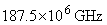(D)  25 GHz

d.   The depth of velocity modulation in a two cavity klystron amplifier can be increased by increasing the

(A)   amplitude of applied signal voltage

(B)   cavity gap transit angle

(C)   catcher cavity dc voltage.

(D)   none of the above.

e.   The semiconductor diode which can be used in switching circuits at microwave range is

(A)     PIN diode                                    (B) Varactor diode

(C)  Tunnel diode                                (D) Gunn diode

f.    In parametric amplifiers used in microwave communication systems, the amplification is limited by

(A)     type of biasing                              (B)  a maximum of 10

(C) pump energy                                 (D)  frequency of operation

g.   A microwave junction is supposed to be matched at all ports, if in the S matrix,

(A)     all the diagonal elements are zero.

(B)     all the diagonal elements are equal but not zero.

(C)     all the diagonal elements are complex.

(D)    is hermitian.

h.   The positions of the probe for half power points in the slotted line of a microwave bench are interpreted to measure

(A)    guide wavelength                          (B) load impedance

(C) source frequency                           (D) high SWR

i.    The main disadvantage of the two-hole directional coupler is

(A)   Poor coupling factor                     (B)  poor directivity

(C) high SWR                                     (D) narrow bandwidth

j.    A PIN diode is frequently used as a

(A)     harmonic generator                       (B) peak clipper

(C)  voltage regulator                           (D) switching diode for frequencies upto

GHz range.

Answer any FIVE Questions out of EIGHT Questions.

Each question carries 16 marks.

Q.2     a.   Define the characteristic impedance of a transmission line. Derive the transmission line equation.                                                                 (8)

b.   A lossless transmission line has a normalised load admittance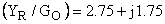.  The observed standing wave ratio on the line is 4.0.  It is proposed to use a short circuited stub to match the load to the line.

(i)                  Determine the electrical distance from the load to the place where the stub should be located.

(ii)                Find the length of the stub in terms of wave length.                      (8)

Q.3     a.   Define the following terms related to directional coupler. Also write S matrix for the same. (i) Directivity (ii) Coupling factor (iii) Insertionloss (iv) Isolation.                                                                   (6)

b.   Mention some of the important features of a slot line and a co-planar line.            (6)

c.   In a TWT, anode voltage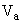=1600 volts, beam current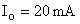, characteristic impedance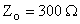, length =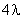, and losses of 3 dB. Find the gain and output.                           (4)

Q.4     a.   Explain the operation of a faraday rotation isolator with the help of neat sketch. List the application of ferrite isolator.                                               (8)

b.   A microstip line of width w = 2 mms is mounted on a quartz substrata  of height h = 0.4 mm.  The dielectric constant of quartz is 3.8 and the loss tangent is 0.0001.  Find the effective permittivity and characteristic impedance of the line at 9GHz.                                                                           (8)

Q.5     a.   List the basic characteristics of a circulator. Discuss any one type with uses.                        (6)

b.   Derive the formula for the attenuation constant of a wave guide in terms of the total power flow along the wave guide and the losses per unit length of the waveguide.                                               (6)

Q.6     a.   How would you measure microwave noise bandwidth and noise factor?              (6)

b.   Determine the transmitter power required in a microwave communication system with the following specifications.

Carrier frequency : 4 GHz.

Minimum power at receiver input : -65 dBw.

Gain of transmitting and receiving antennas : 45 dB

Distance between antennas : 60 Km.

If the distance between the antenna is doubled, what happens to the power loss.                 (6)

Q.7     a.   Explain the principle and operation of two-cavity klystron with a neat sketch.                      (8)

b.  How does the function of the magnetic field in a TWT differ from its function in a magnetron?                                                                      (4)

c.   A Gunn diode is working in transit time modes at 12 GHz. The domain of charges move at 107 cm/sec speed. Calculate the length of the device. Can the device work at 14 GHz? If Yes, what will be the mode of operation?                                           (4)

Q.8     a.   Explain the principles of operation of TRAPATT diode with the help of voltage and current waveforms.                                                                 (8)

b.   Two cavity klystron has the following parameters. Beam voltage               V0 = 20 KV, operating frequency  f = 10 GHz, Beam current Z0 = 10 GHz, Beam comling coefficient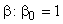, dc electron beam current density = 10-8 c/m3, signal voltage Vi=- 10 V (rms) shunt resistance of cavity Rsh=15 k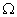, total shunt resistance including load R = 35 k. Find (i) Plasma frequency (ii) the induced current at the output cavity (iii) Power gain (iv) electronic efficiency.                                                                             (8)

Q.9          Write short notes on any TWO of the following:

(i)                  Measurement of microwave power.

(ii)                Reflex Klystron.

(iii)               Duct Propagation.

(iv)              Line of sight communications.   (2 x 8)